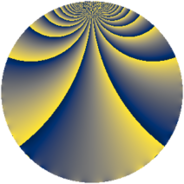# Properties

 Label 1815.2.uLevel $1815$ Weight $2$ Character orbit 1815.u Rep. character $\chi_{1815}(166,\cdot)$ Character field $\Q(\zeta_{11})$ Dimension $880$ Sturm bound $528$

# Related objects

## Defining parameters

 Level: $$N$$ $$=$$ $$1815 = 3 \cdot 5 \cdot 11^{2}$$ Weight: $$k$$ $$=$$ $$2$$ Character orbit: $$[\chi]$$ $$=$$ 1815.u (of order $$11$$ and degree $$10$$) Character conductor: $$\operatorname{cond}(\chi)$$ $$=$$ $$121$$ Character field: $$\Q(\zeta_{11})$$ Sturm bound: $$528$$

## Dimensions

The following table gives the dimensions of various subspaces of $$M_{2}(1815, [\chi])$$.

Total New Old
Modular forms 2680 880 1800
Cusp forms 2600 880 1720
Eisenstein series 80 0 80

## Trace form

 $$880q + 8q^{2} - 80q^{4} + 8q^{7} + 24q^{8} + 880q^{9} + O(q^{10})$$ $$880q + 8q^{2} - 80q^{4} + 8q^{7} + 24q^{8} + 880q^{9} + 4q^{10} + 16q^{11} - 28q^{13} - 56q^{14} - 64q^{16} + 24q^{17} + 8q^{18} + 8q^{19} + 28q^{22} - 64q^{23} + 24q^{24} - 88q^{25} + 8q^{26} + 48q^{28} + 48q^{29} + 4q^{30} - 20q^{31} + 96q^{32} + 4q^{33} + 72q^{34} + 8q^{35} - 80q^{36} + 40q^{37} + 56q^{38} + 8q^{39} - 120q^{40} + 32q^{41} + 24q^{42} + 40q^{43} + 28q^{44} + 64q^{46} + 32q^{47} + 32q^{48} - 84q^{49} + 8q^{50} + 16q^{51} - 156q^{52} - 176q^{53} + 8q^{55} + 52q^{56} + 8q^{57} - 68q^{58} + 40q^{59} + 80q^{61} - 164q^{62} + 8q^{63} - 72q^{64} + 24q^{65} + 40q^{66} - 20q^{67} + 80q^{68} + 8q^{69} + 24q^{70} + 40q^{71} + 24q^{72} + 20q^{73} + 96q^{74} - 12q^{76} + 88q^{77} - 156q^{78} - 132q^{79} + 880q^{81} + 48q^{82} + 56q^{83} + 72q^{84} + 8q^{85} + 88q^{86} + 32q^{87} + 92q^{88} - 80q^{89} + 4q^{90} - 36q^{91} + 136q^{92} + 16q^{93} - 32q^{94} - 176q^{96} - 100q^{97} - 256q^{98} + 16q^{99} + O(q^{100})$$

## Decomposition of $$S_{2}^{\mathrm{new}}(1815, [\chi])$$ into newform subspaces

The newforms in this space have not yet been added to the LMFDB.

## Decomposition of $$S_{2}^{\mathrm{old}}(1815, [\chi])$$ into lower level spaces

$$S_{2}^{\mathrm{old}}(1815, [\chi]) \cong$$ $$S_{2}^{\mathrm{new}}(121, [\chi])$$$$^{\oplus 4}$$$$\oplus$$$$S_{2}^{\mathrm{new}}(363, [\chi])$$$$^{\oplus 2}$$$$\oplus$$$$S_{2}^{\mathrm{new}}(605, [\chi])$$$$^{\oplus 2}$$